# SSAT Upper Level Math : How to multiply

## Example Questions

### Example Question #21 : Basic Addition, Subtraction, Multiplication And Division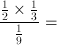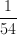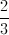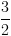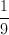Explanation:

First multiply the fraction in the numerator.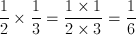Now we have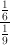Never divide fractions.  We multiply the numerator by the reciprocal of the denominator.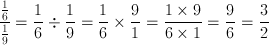### Example Question #22 : Basic Addition, Subtraction, Multiplication And Division

Which of these expressions is the greatest?

Twenty percent of one fourth

All of these expressions are equivalent

Twenty-five percent of one fifth

One fifth of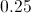One fourth of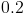All of these expressions are equivalent

Explanation:

The easiest way to see that all four are equivalent is to convert each to a decimal product, noting that twenty percent and one-fifth are equal to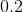, and twenty-five percent and one fourth are equal to.

One fourth of 0.2: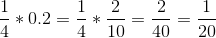One fifth of 0.25: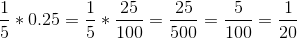Twenty-five percent of one fifth: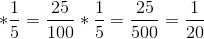Twenty percent of one fourth: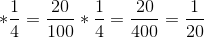### Example Question #1 : How To Multiply

Write .007341 in scientific notation.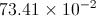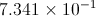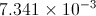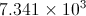Explanation:

The answer is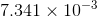### Example Question #2 : How To Multiply

If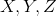are consecutive negative numbers, which of the following is false?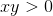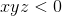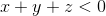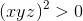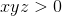Explanation:

When three negative numbers are multiplied together, the product will be negative as well. All the other expressions are true.

### Example Question #25 : Basic Addition, Subtraction, Multiplication And Division

Fill in the circle to yield a true statement: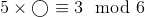Explanation:

The problem is asking for a number whose product with 5 yields a number congruent to 3 in modulo 6 arithmetic - that is, a number which, when divided by 6, yields remainder 3. We multiply 5 by each choice and look for a product with this characteristic.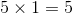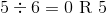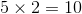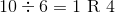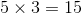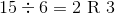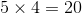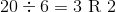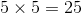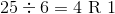The only choice whose product with 5 yields a number congruent to 3 modulo 6 is 3, so this is the correct choice.

### Example Question #26 : Basic Addition, Subtraction, Multiplication And Division

You are asked to fill in all three circles in the statement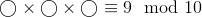with the same number from the set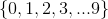to make a true statement.

How many ways can you do this?

One

Five

Two

Four

Three

One

Explanation:

The problem is asking for a number whose cube is a number congruent to 9 in modulo 10 arithmetic - that is, a number whose cube, when divided by 10, yields remainder 9. If the quotient of a number and 10 has remainder 9, then it is an integer that ends with the digit "9". Since this makes the cube odd, the number that is cubed must also be odd, so we need only test the five odd integers: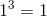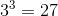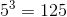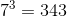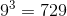Only 9 fits the criterion, so "one" is the correct response.

### Example Question #27 : Basic Addition, Subtraction, Multiplication And Division

You are asked to fill in both circles in the statement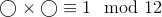with the same number from the set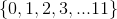to make a true statement.

How many ways can you do this?

None

Six

None of the other responses is correct.

Two

Four

Four

Explanation:

The problem is asking for a number whose square is a number congruent to 1 in modulo 12 arithmetic - that is, a number whose square, when divided by 12, yields remainder 1. This square must be odd, so the number squared must also be odd. Therefore, we need only test the odd integers. We see that: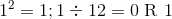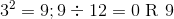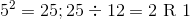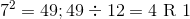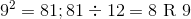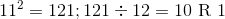Four of the integers have squares congruent to 1 in modulo 12 arithmetic.

### Example Question #28 : Basic Addition, Subtraction, Multiplication And Division

Multiply: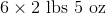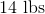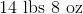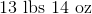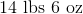None of the other responses is correct.Explanation:

We can write 2 pounds, 5 ounces as just ounces as follows: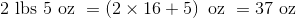Multiply: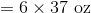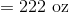Divide by 16, noting quotient and remainder, to get pounds and ounces: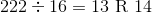Therefore, the correct response is 13 pounds, 14 ounces.

### Example Question #31 : Basic Addition, Subtraction, Multiplication And Division

Fill in the circle to yield a true statement: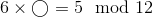The other answer choices are incorrect.The other answer choices are incorrect.

Explanation:

The problem is asking for a number whose product with 6 yields a number congruent to 5 in modulo 12 arithmetic - that is, a number which, when divided by 12, yields remainder 6.

However, 6 multiplied by any odd number yields a number which, when divided by 12, yields remainder 6, as is demonstrated using our choices: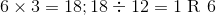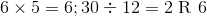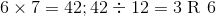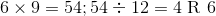Each of the choices yields a product congruent to 6 modulo 12, so none of them is a correct choice.

### Example Question #3 : How To Multiply

Write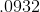in scientific notation.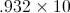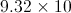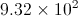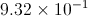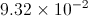The given number has three significant figure (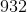) so we write out number as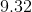You must move the decimal place two places to the right, or in other words multiply by. When you move the decimal place to the right, you multiply the number by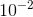, so it is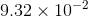.